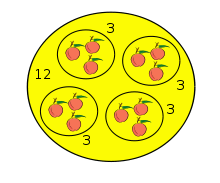# Quotient

For other uses, see Quotient (disambiguation).
Calculation resultsSubtraction (−)Multiplication (×)Division (÷)Modulo (mod)Exponentiationnth root (√)Logarithm (log)The quotient of 12 apples by 3 apples is 4.

In arithmetic, a quotient (from Latin: quotiens "how many times", pronounced ˈkwoʊʃənt) is the result of division. For example, when dividing twenty-one (the dividend) by three (the divisor), the quotient is seven.

The quotient may sometimes be defined as the number of times the divisor may be subtracted from the dividend without the remainder becoming negative. For example, the divisor 3 may be subtracted up to 7 times from the dividend 21 before the remainder becomes negative: 21-3-3-3-3-3-3-3 = 0.

Outside of arithmetic, many branches of math have co-opted the word "quotient" to describe structures built by breaking larger structures into pieces. Given a set with an equivalence relation defined on it, a "quotient set" may be created which contains those equivalence classes as elements. A quotient group may be formed by breaking a group into a number of similar cosets, while a quotient space may be formed in a similar process by breaking a vector space into a number of similar linear subspaces.